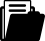Meine Position
i
Ein Tipp vom Eldar Team
Je mehr Sie bestellen, desto grösser Ihr RabattBuch
Buch
Fachbuch
2009

# Mathematical Methods in Robust Control of Discrete-Time Linear Stochastic Systems

ISBN
EAN
978-1-4419-0629-8
9781441906298
Artikel-Nr.
LKZ23QG
Sofort lieferbar:
3
Kostenloser Versand
CHF
166.05
Anzahl
1
Maximale
Lieferzeit
5
Arbeitstage
Montag
27.09.2021Beschreibung

In this monograph the authors develop a theory for the robust control of discrete-time stochastic systems, subjected to both independent random perturbations and to Markov chains. Such systems are widely used to provide mathematical models for real processes in fields such as aerospace engineering, communications, manufacturing, finance and economy. The theory is a continuation of the authors’ work presented in their previous book entitled "Mathematical Methods in Robust Control of Linear Stochastic Systems" published by Springer in 2006.

Key features:

- Provides a common unifying framework for discrete-time stochastic systems corrupted with both independent random perturbations and with Markovian jumps which are usually treated separately in the control literature;

- Covers preliminary material on probability theory, independent random variables, conditional expectation and Markov chains;

- Proposes new numerical algorithms to solve coupled matrix algebraic Riccati equations;

- Leads the reader in a natural way to the original results through a systematic presentation;

- Presents new theoretical results with detailed numerical examples.

The monograph is geared to researchers and graduate students in advanced control engineering, applied mathematics, mathematical systems theory and finance. It is also accessible to undergraduate students with a fundamental knowledge in the theory of stochastic systems.

Inhaltsverzeichnis
Elements of probability theory.- Discrete-time linear equations defined by positive operators.- Mean square exponential stability.- Structural properties of linear stochastic systems.- Discrete-time Riccati equations of stochastic control.- Linear quadratic optimization problems.- Discrete-time stochastic optimal control.- Robust stability and robust stabilization of discrete-time linear stochastic systems.Herausgeber/-innen
Zielgruppe
Research
Leserkritik

From the reviews:

“This monograph deals with the control theory of linear discrete-time stochastic systems subject to multiplicative white noise and to Markov jumping, as arising in many engineering areas, such as communications, fault detection and isolation, robust control, stochastic filtering navigation as wells as in finance, economics and biology. … The theoretical developments are illustrated by several examples. … The book is suitable for advanced courses in robust control of discrete-time stochastic systems, where a good knowledge of probability theory and linear control systems is needed.” (Kurt Marti, Zentralblatt MATH, Vol. 1183, 2010)

“The book is dedicated to control theory of linear discrete-time stochastic systems perturbed by both multiplicative white noise and Markov jumps. … The book is recommended to graduate students and researchers in the field of applied mathematics and stochastic control.”­­­ (Pavel Pakshin, Mathematical Reviews, Issue 2011 d)

Publikation
Vereinigte Staaten
24.11.2009Sprache
EnglischFormat
Hardcover
346 SeitenWeitere FormateVersand
Kostenloser Versand: Schweiz & Liechtenstein
Für den Versand nach Deutschland oder Frankreich werden die Versandgebühren der Schweizerischen Post berechnet. Diese werden Ihnen im Warenkorb für Ihre gesamte Bestellung berechnet.Themen
Zurück
Zum Start